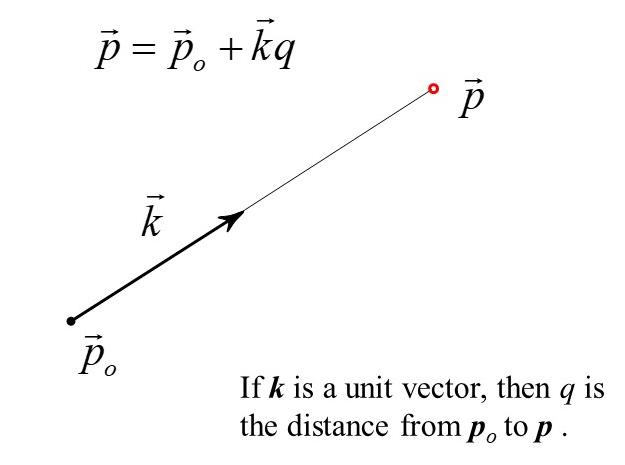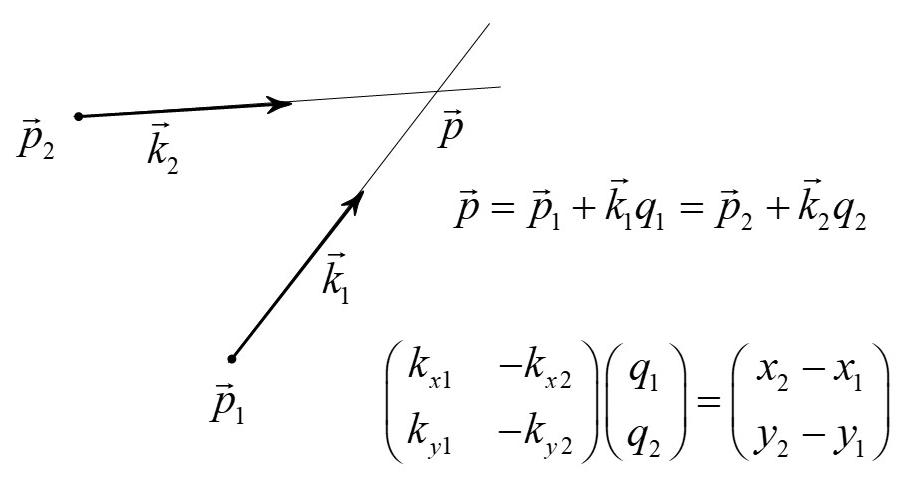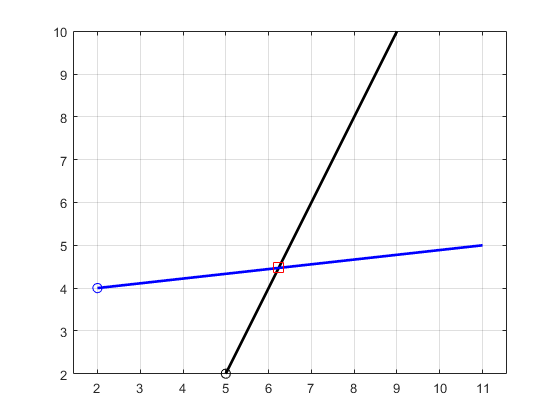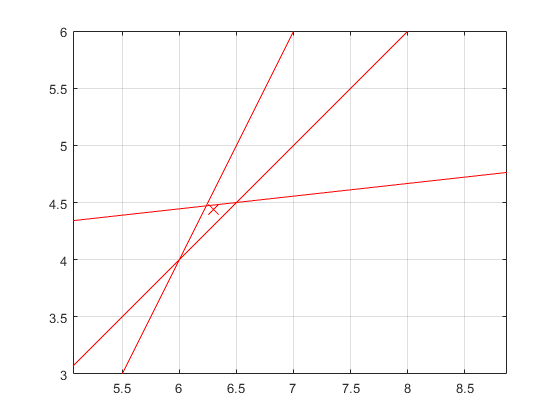# Intersecting Lines

A line can be defined parametricallyThe shortest distance from a point to a line is the perpendicular vector dThe intersection of two parametric lines can be found fromSee perp.m for example code.

The intersection of more than two lines can be found by minimizing the sum of the squared distances from the solution point to each of the intersecting lines.
The method is given in intersect_lines.mSee perp_script3.m for this example

Maintained by John Loomis, last updated 21 January 2020